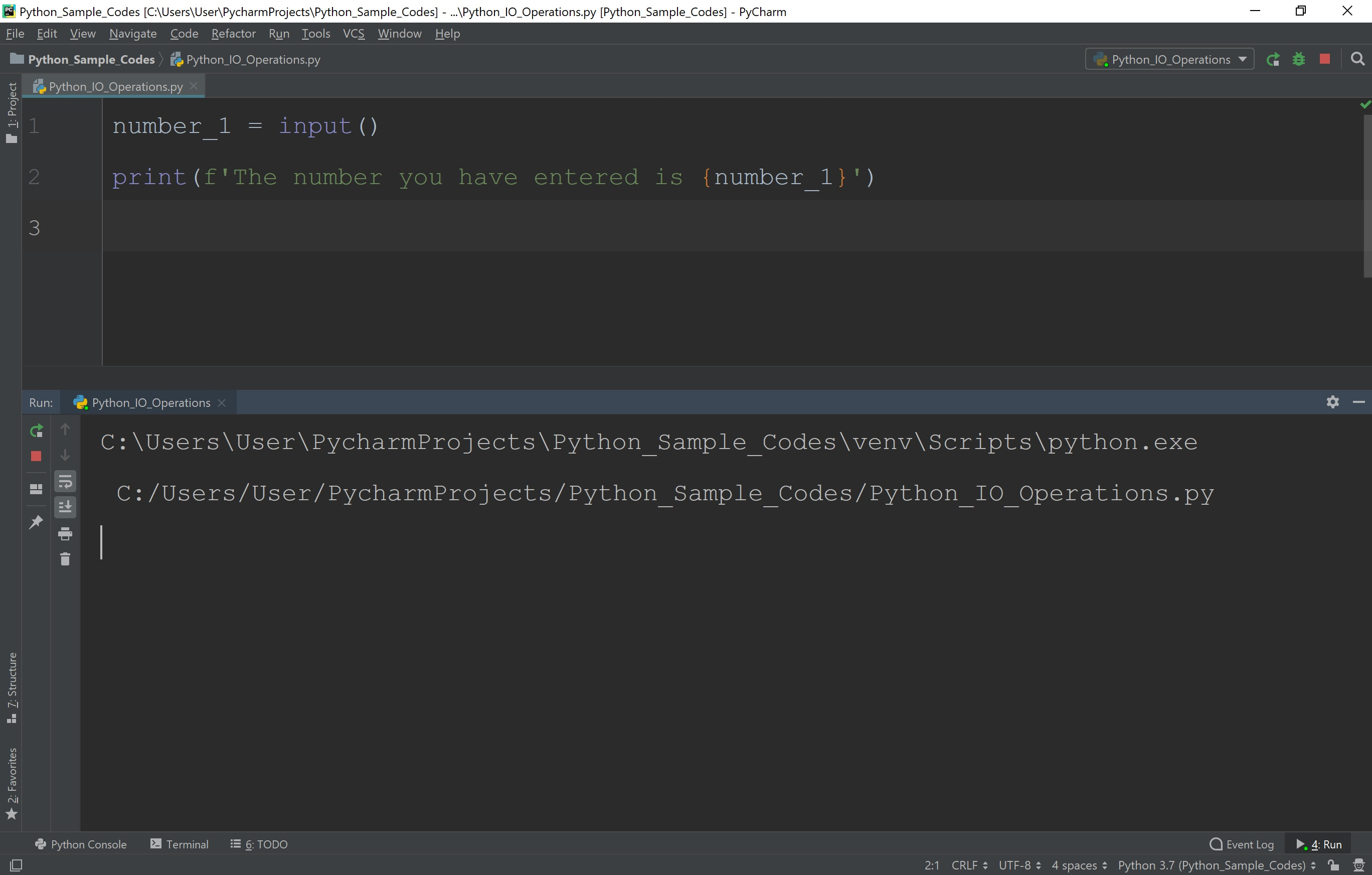The perfect place for easy learning...

×

# Python IO Operations

In Python, input and output operations (OI Operations) are performed using two built-in functions. Following are the two built-in functions to perform output operations and input operations.

• print( ) - Used for output operation.
• input( ) - Used for input operations.

## Output Operations using print() function

The built-in function print( ) is used to output the given data to the standard output device (Screen).

## Displaying a message using print( ) function

In Python, using the print( ) function we can display a text message. The print( ) takes a string as an argument and displays the same.

# Example

``````
print('This message is displayed on the screen')

print("This message is also displayed on the screen")
```
```

When we use the print( ) function to display a message, the string can be enclosed either in the single quotation or double quotation.

## Displaying a variable value using print( ) function

In Python, the built-in function print( ) function is also used to display variables value. The following example shows that how to display variable value using print( ) function.

# Example

``````
num1 = 10   #variale num1 with value 10
print(num1)

#Output : 10
```
```

In the above example, we have created a variable num1 with a value 10. The value of the variable num1 is displayed using the print( ) function.

## Formatted print( ) function to display a combination of message and value

The built-in function print( ) is also used to display the combination of message and variable value. Let's look at the following example.

# Example

``````
num1 = 10   #variale num1 with value 10
print('The value of variable num1 is ' + num1)

#Output : The value of variable num1 is 10
```
```

We can also write the same print( ) function as follows.

# Example

``````
num1 = 10   #variale num1 with value 10
print(f"The value of variable num1 is {num1}")

#Output : The value of variable num1 is 10
```
```

In the above example code, we have used a formatted string. In Python, we can use the formatted string that is prefixed with character "f". In the formatted string, the variable values are included using curly braces ({ }).

Always the return value of print( ) function is None.

## Input Operations using input() function

The Python provides a built-in function input( ) to perform input operations. The input( ) function can be used either with some message or without a message.

When input( ) function is used without a message, it simply prompts for the input value. When the user enters the input value it reads the entered value as a string and assigns it to the left-hand-side variable.

# Example

``````
number_1 = input()
print(f'The number you have entered is {number_1}')
```
```

When we run the above piece of code, in the output screen simply it waits for the user input without displaying anything. Observe the following figure.Here, the major problem is that the user does not have any information regarding what is the next step the user has to do? To solve this problem, we use the input( ) function with a message which tells the user that what the user has to do?

When input( ) function is used with a message, it prompts with a given message for the input value. When the user enters the input value it reads the entered value as a string and assigns it to the left-hand-side variable.

# Example

``````
number_1 = input('Enter any number: ')
print(f'The number you have entered is {number_1}')
```
```

When we run the above piece of code, in the output screen is displayed with a given message and waits for the user input. Observe the following figure.Always the input( ) function reads input value as string value only.
To read the value of any other data type, there is no input function in Python. Explicitly we need to convert to the required data type using type casing.

## Reading a single character in Python

The Python does not provide any direct function to read a single character as input. But we can use the index value to extract a single character from the user entered input value. Let's look at the following Python code.

# Example

``````
ch = input('Enter any data: ')
print(f'The character entered is {ch}')
```
```

When we run the above piece of code, the input( ) function reads complete data entered by the user but assigns an only first character to the variable ch. Look at the following figure.In the above example, the user has entered 'abc' as input value but the variable ch is assigned with value 'a' only. Similarly, we can extract any character form the input data using the index value.